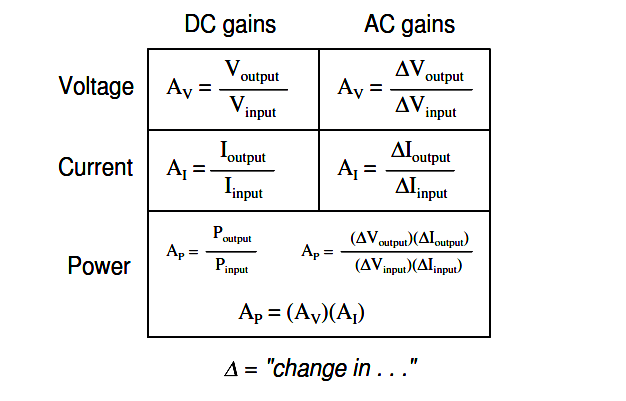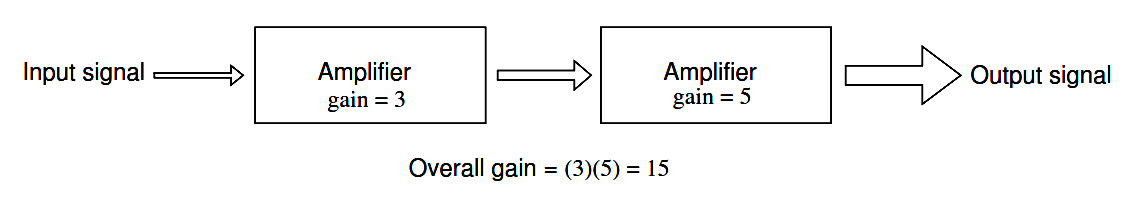# Ampliﬁer Gain

You are here:

## Voltage Gain

Because ampliﬁers have the ability to increase the magnitude of an input signal, it is useful to be able to rate an ampliﬁer’s amplifying ability in terms of an output/input ratio.

The technical term for an ampliﬁer’s output/input magnitude ratio is gain. As a ratio of equal units (power out / power in, voltage out / voltage in, or current out / current in), gain is naturally a unitless measurement. Mathematically, gain is symbolized by the capital letter “A”.

## How to Calculate Voltage Gain?

For example, if an ampliﬁer takes in an AC voltage signal measuring 2 volts RMS and outputs an AC voltage of 30 volts RMS, it has an AC voltage gain of 30 divided by 2, or 15:

AV = Voutput / Vinput

AV = 30 / 2

AV = 15

Correspondingly, if we know the gain of an ampliﬁer and the magnitude of the input signal, we can calculate the magnitude of the output.

For example, if an ampliﬁer with an AC current gain of 3.5 is given an AC input signal of 28 mA RMS, the output will be 3.5 times 28 mA, or 98 mA:

Ioutput = (AI)(Iinput)

Ioutput = (3.5)(28 mA)

Ioutput = 98 mA

In the last two examples, I speciﬁcally identiﬁed the gains and signal magnitudes in terms of “AC.” This was intentional, and illustrates an important concept: electronic ampliﬁers often respond differently to AC and DC input signals and may amplify them to different extents.

Another way of saying this is that ampliﬁers often amplify changes or variations in input signal magnitude (AC) at a different ratio than steady input signal magnitudes (DC).

The speciﬁc reasons for this are too complex to explain at this time, but the fact of the matter is worth mentioning. If gain calculations are to be carried out, it must ﬁrst be understood what type of signals and gains are being dealt with, AC or DC.

## Amplifier Gains

Electrical ampliﬁer gains may be expressed in terms of voltage, current, and/or power, in both AC and DC.

A summary of gain deﬁnitions is as follows.

The triangle-shaped “delta” symbol (∆) represents change in mathematics, so “∆Voutput / ∆Vinput ” means “change in output voltage divided by change in input voltage,” or more simply, “AC output voltage divided by AC input voltage”:If multiple ampliﬁers are staged, their respective gains form an overall gain equal to the product (multiplication) of the individual gains. (Figure 1.6) If a 1 V signal were applied to the input of the gain of 3 ampliﬁer in Figure 1.6 a 3 V signal out of the ﬁrst ampliﬁer would be further ampliﬁed by a gain of 5 at the second stage yielding 15 V at the ﬁnal output.Figure 1.6: The gain of a chain of cascaded ampliﬁers is the product of the individual gains.